# Multi-valued mapping

A mapping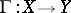associating with each elementof a seta subset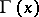of a set. If for eachthe setconsists of one element, then the mappingis called single-valued. A multi-valued mappingcan be treated as a single-valued mapping ofinto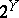, that is, into the set of all subsets of.
For two multi-valued mappings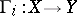,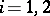, their inclusion is naturally defined: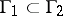if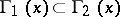for all. For any family of multi-valued mappings,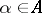, the union and intersection are defined: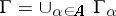iffor alland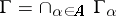if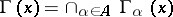for all. For any family of multi-valued mappings,, the multi-valued mappingis called the Cartesian product of the multi-valued mappings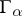if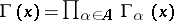. A section of a multi-valued mappingis a single-valued mappingsuch thatfor all. The graph of a multi-valued mappingis the set.
A multi-valued mappingof a topological spaceinto a topological spaceis called upper semi-continuous if for every open set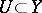the set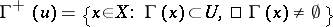is open in, or equivalently: For anyand any neighbourhoodofthere is a neighbourhood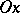ofsuch that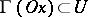, where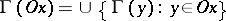. A multi-valued mapping from a topological spaceto a topological spaceis called lower semi-continuous if for any open setthe set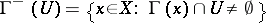is open in. If a multi-valued mapping satisfies both properties simultaneously, then it is called a continuous multi-valued mapping.
Letbe a topological vector space. A multi-valued mappingis called convex-compact valued ifis a convex compact set for all. For a finite set of multi-valued mappings,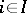, the algebraic sumis defined by. The intersection of any (finite) family of upper semi-continuous (respectively, continuous) multi-valued mappings is upper semi-continuous (respectively, continuous). The Cartesian product of a finite family of upper semi-continuous multi-valued mappings is upper semi-continuous. The algebraic sum of a finite family of upper semi-continuous (convex-compact valued) mappings is upper semi-continuous (convex-compact valued). The intersection and Cartesian product of any family of convex-compact valued mappings is convex-compact valued.
Letbe a paracompact space anda locally convex metric linear space (cf. Locally convex space; Linear space; Metric space). Letbe a multi-valued mapping which is upper semi-continuous and is such thatis closed infor every. Then the multi-valued mappingadmits continuous sections. Letand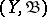be spaces with given-algebrasand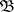; a multi-valued mappingis called measurable if the graph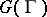belongs to the smallest-algebra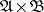of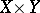containing all sets of the form, where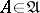and. Ifis a measurable multi-valued mapping from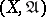to a complete separable metric space, whereis the Borel-algebra of, thenhas measurable sections.